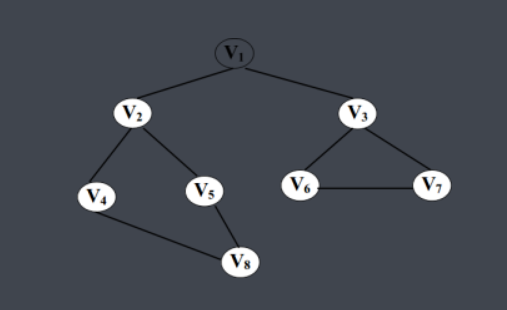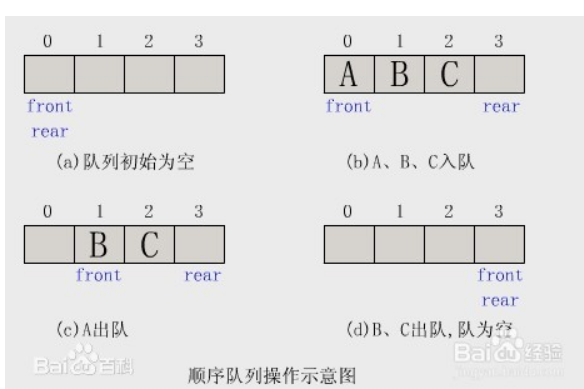## 广度优先搜索bfs

本某电子科技大学计算机专业的菜鸡立个Flag，从现在开始自学，希望大二的时候能入acm的门，给各位大佬端茶倒水。现在开始记录第一篇有关广度优先算法的博客。```typedef struct{
int x;//节点的横纵坐标
int y;
}NODE;```

NODE a；

queue<NODE> Q;

1、Q.pop();//弹出队列中最前面的一个元素，即最早进入的那个元素，让他消失。

2、Q.front();//取出队列中最前面的一个元素的值。

3、Q.empty();//判断队列是否为空。

4、Q.push(a);//将一个相应类型的元素压入队列中。

```void bfs(int x,int y,int max) {

node s,p,b;
s.x=x;
s.y=y;
N.push(s);//将起始元素压入队列
visit[x][y]=true;//访问过了起始元素，标记一下，以免重复访问
while(!N.empty()) {
p = N.front();//取出队列最前面的那个元素，以他为新的支点找相邻的可经一步转移达到的状态
N.pop();
for(i=0; i<4; i++) {//原题是有上下左右四个方向可走，所以这里即搜索相邻的四个方向
b.x=p.x+pos[i];//对横纵坐标的变换处理
b.y=p.y+pos[i];
if(valid(b.x,b.y,max)&&!visit[b.x][b.y]) {//判断是否满足入队条件，即未被访问过，未越界
N.push(b);
visit[b.x][b.y]=true;//访问过了节点元素，标记一下
}

}
}

}```